NCERT Solutions: Heron’s Formula (Exercise 10.1)

# Heron’s Formula (Exercise 10.1) NCERT Solutions - Mathematics (Maths) Class 9

Q1. A traffic signal board, indicating ‘SCHOOL AHEAD’, is an equilateral triangle with side ‘a’. Find the area of the signal board, using Heron’s formula. If its perimeter is 180 cm, what will be the area of the signal board?
Ans: For an equilateral triangle with side ‘a’, area = (√3/4) a2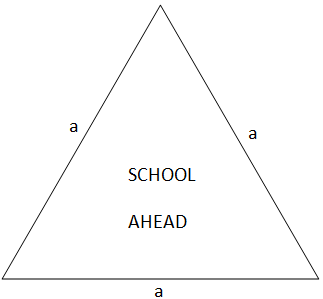∵ Each side of the triangle = a cm

Perimeter of the signal board will be
∴ a + a + a = 180 cm
3a = 180 cm
a= (180/3) = 60 cm
Now, s = Semi-perimeter = (180/2) = 90 cm
∵ Area of triangle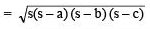Area of the given triangle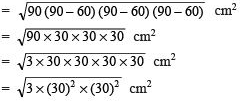= 30 x 30 x√3 = 900√3 cm2
Thus, the area of the given triangle = 900√3cm2.

Q2. The triangular side walls of a flyover have been used for advertisements. The sides of the walls are 122 m, 22 m and 120 m (see Fig). The advertisements yield an earning of ₹ 5000 per m2 per year. A company hired one of its walls for 3 months. How much rent did it pay?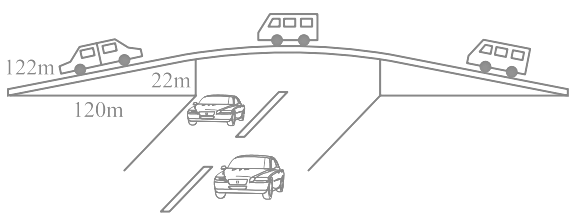Ans: The sides of the triangular wall are a = 122 m, b = 120 m, c = 22 m.

Now, the perimeter will be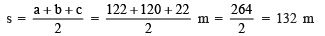∵ The area of a triangle is given by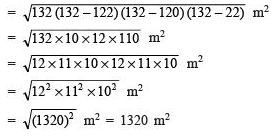∵ Rent for 1 year (i.e. 12 months) per m2 = Rs. 5000
∴ Rent for 3 months per m2 = Rs. 5000 x (3/12)
⇒ Rent for 3 months for 1320 m2 = 5000 x (3/12) x 1320 = 5000 x 3 x 110 = Rs.16,50,000.

Q3. There is a slide in a park. One of its side walls has been painted in some colour with a message “KEEPTHE PARK GREEN AND CLEAN” (see Fig). If the sides of the wall are 15 m, 11 m and 6 m, find the area painted in colour.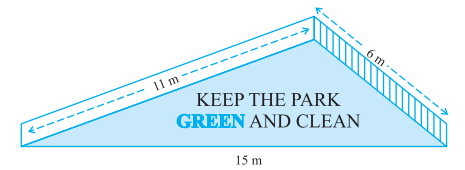Ans: Sides of the wall a = 15 m, b = 11 m, c = 6 m.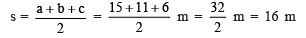The area of the triangular surface of the wall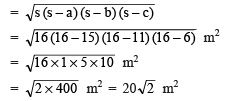Thus, the required area painted in colour = 20√2 m2.

Q4. Find the area of a triangle, two sides of which are 18 cm and 10 cm and the perimeter is 42 cm.
Ans: Let the sides of the triangle be a = 18 cm, b = 10 cm and c =?
∵ Perimeter (2s) = 42 cm
s = (42/2)  = 21 cm
c = 42 – (18 + 10) cm = 14 cm
∵ Area of a triangle =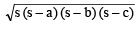∴ Area of the given triangle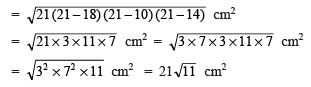Thus, the required area of the triangle = 21√11 cm2.

Q5. Side of a triangle is in the ratio of 12: 17: 25 and its perimeter is 540 cm. Find its area.
Ans: The perimeter of the triangle = 540 cm.
Semi-perimeter of the triangle, s= (540/2) = 270 cm
∵ The sides are in the ratio of 12: 17: 25.
∴ Let, the side be a = 12x cm, b = 17x cm, c = 25x cm.
12x + 17x + 25x = 540
54x = 540
x = (540/54) = 10
∴ a = 12 x 10 = 120 cm, b = 17 x 10 = 170 cm, c = 25 x 10 = 250 cm.
(s – a) = (270 – 120) cm = 150 cm
(s – b) = (270 – 170) cm = 100 cm
(s – c) = (270 – 250) cm = 20 cm
∴ Area of the triangle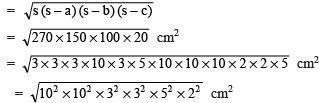= 10 x 10 x 3 x 3 x 5 x 2 cm2 = 9,000 cm2
Thus, the required area of the triangle = 9,000 cm2.

Q6. An isosceles triangle has a perimeter of 30 cm and each of the equal sides is 12 cm. Find the area of the triangle.
Ans: Equal sides of the triangle are 12 cm each.
Let the third side = x cm.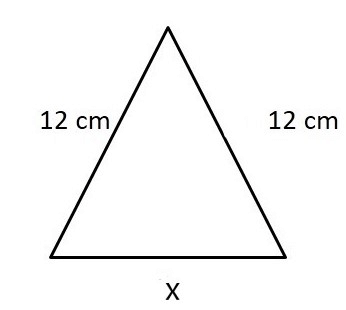∵ Perimeter = 30 cm
∴ 12 cm + 12 cm + x cm = 30 cm
x = 30 – 12 – 12 = 6 cm
Semi-perimeter = (30/2) cm = 15 cm
∴ Area of the triangle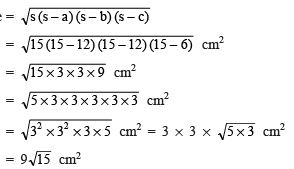Thus, the required area of the triangle = 9√15 cm2.

The document Heron’s Formula (Exercise 10.1) NCERT Solutions | Mathematics (Maths) Class 9 is a part of the Class 9 Course Mathematics (Maths) Class 9.
All you need of Class 9 at this link: Class 9

## Mathematics (Maths) Class 9

42 videos|378 docs|65 tests

## FAQs on Heron’s Formula (Exercise 10.1) NCERT Solutions - Mathematics (Maths) Class 9

 1. What is Heron's formula?Ans. Heron's formula is a method used to find the area of a triangle when the lengths of all three sides are known.
 2. How is Heron's formula derived?Ans. Heron's formula is derived using the semi-perimeter of the triangle and the lengths of its sides. It is based on Heron's theorem, which states that the area of a triangle can be calculated using the semi-perimeter and the lengths of its sides.
 3. Can Heron's formula be used for all types of triangles?Ans. Yes, Heron's formula can be used to find the area of any type of triangle, including equilateral, isosceles, and scalene triangles.
 4. What are the steps to use Heron's formula?Ans. The steps to use Heron's formula are as follows: 1. Calculate the semi-perimeter of the triangle by adding the lengths of all three sides and dividing by 2. 2. Use the semi-perimeter and the lengths of the sides in Heron's formula: Area = √(s(s-a)(s-b)(s-c)), where s is the semi-perimeter and a, b, and c are the lengths of the sides. 3. Simplify the equation and calculate the square root to find the area of the triangle.
 5. Can Heron's formula be used to find the lengths of the sides of a triangle?Ans. No, Heron's formula is used only to find the area of a triangle. It cannot be used to find the lengths of the sides of a triangle.

## Mathematics (Maths) Class 9

42 videos|378 docs|65 testsExplore Courses for Class 9 examSignup to see your scores go up within 7 days! Learn & Practice with 1000+ FREE Notes, Videos & Tests.
10M+ students study on EduRev
Track your progress, build streaks, highlight & save important lessons and more!
Related Searches

,

,

,

,

,

,

,

,

,

,

,

,

,

,

,

,

,

,

,

,

,

;